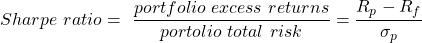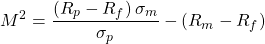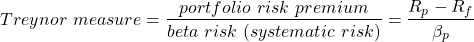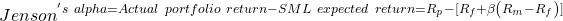Concept 66: Applications of the CAPM and the SML | IFT World
101 Concepts for the Level I Exam

# Concept 66: Applications of the CAPM and the SML• Expected return of an asset will be equal to the required return on the asset for a market in equilibrium.
• SML can be used to find overvalued and undervalued securities.
• Securities on the SML lineà fairly valued,
• Securities above the SML line à undervalued,
• Securities below the SML line à

The market has an expected return of 16% and a risk-free rate of 5%. Compute the expected and required return on each stock and determine whether they are fairly valued, overvalued or undervalued. The following are the street forecasts for the stocks:

 Security Current price Expected year-end price Expected dividend Beta X $10$10.5 $0.5 0.7 Y$200 $226.0$6.0 1.0 Z $16$18.0 \$2.0 1.3

Solution:

 Security Expected or forecasted return Required return Valuation X (10.5 – 10 + 0.5)/(10) = 10.0% 0.05 + 0.7 (0.16 – 0.05) = 12.7% Overvalued Y (226 – 200 + 6)/(200) = 16.0% 0.05 + 1.0 (0.16 – 0.05) = 16.0% Fairly valued Z (18 – 16 + 2)/(16) = 25.0% 0.05 + 1.3 (0.16 – 0.0 5) = 19.3% Undervalued

Appropriate strategy:

• Sell X.
• Ignore Y.

The four measures commonly used in performance evaluation are:

1. Sharpe ratio: is the slope of CML and CAL line.2. M-Squared produces same portfolio ranking as that of Sharpe ratio, but is stated in percentage terms.Intuition: The portfolio excess returns have been levered so that it has the same risk as that of the market (factor used is (). This is then compared with the market risk premium.

Note: Portfolios that lie above the CML line have greater Sharpe ratios than the CML portfolios and positive M2 values.

3. Treynor measure is slope of lines, similar to the Sharpe ratio, but for systematic risk.4. Jenson’s alpha is similar to M2, but for systematic risk. It gives percentage return in excess of the expected return from SML.Note: Portfolios that lie above the SML line have greater Treynor measures than the SML portfolios and positive Jenson’s alpha values.